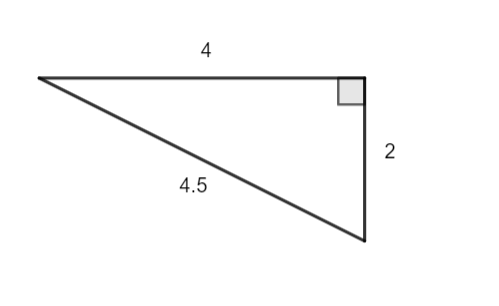Area of a Triangle homework Help at TutorEye

# Best Homework Help For Area of a Triangle

## Top Questions

t schedule 3 people for the day my % scheduled is 78.57% My question is how did the computer come up with the 78.57%?
View More

I would love to get a written solution. I did the other proof tree that you can see in the attaached files. So its supposed to look like that Thank You.
View More

xtbook. i really need in in half an hour
View More

## Area of a Triangle:

The area of an object would be the amount of space covered by its flat surface. Hence, the area of a triangle would be space occupied within its three sides. The area is measured under the metric system of units as square centimeters cm2, square meters m2.

The formula used to compute the area of a triangle is. A=(1/2)bh.

Where b is the base of the triangle, and h is the height of the triangle.

## Area of a Triangle Sample Questions:

Question 1: Find the area: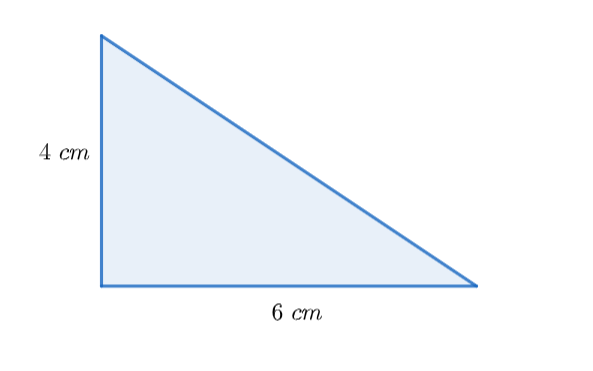Explanation: The area would be, A=(1/2)bh.

Question 2: Compute the area: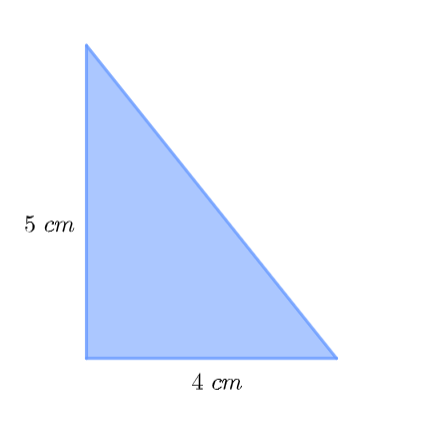Explanation: The area would be, A=(1/2)bh.

Question 3: Find the area of the shape.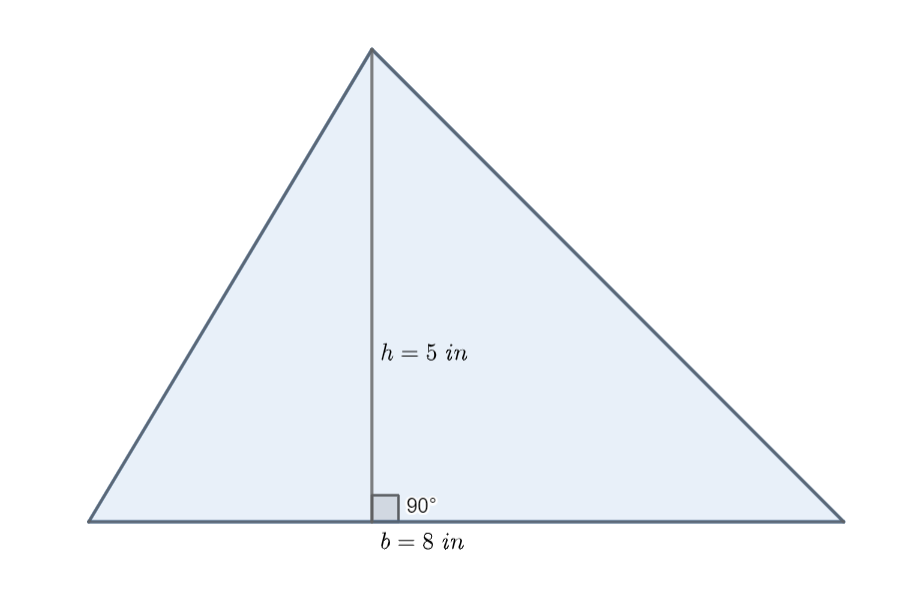Explanation: The area would be, A=(1/2)bh.

Question 4: Find the area of the shape.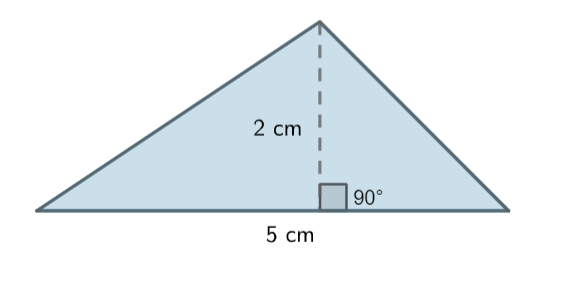Explanation: The area would be, A=(1/2)bh.

Question 5: The following triangle has an area of 16 square units. Find the missing side.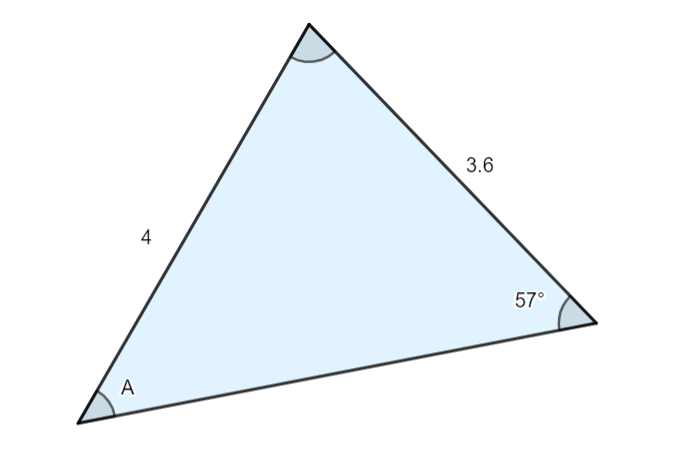Explanation: The area covered by the object would be, A=(1/2)bh.

Question 6: The following triangle has an area of 6 square units. Find the missing side.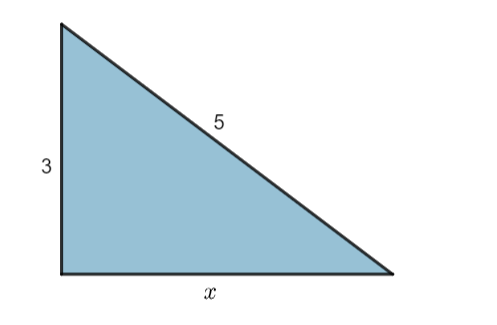Explanation: The area covered by the object would be, A=(1/2)bh.

Question 7: The following figure has an area of 9 square units. Find the missing side.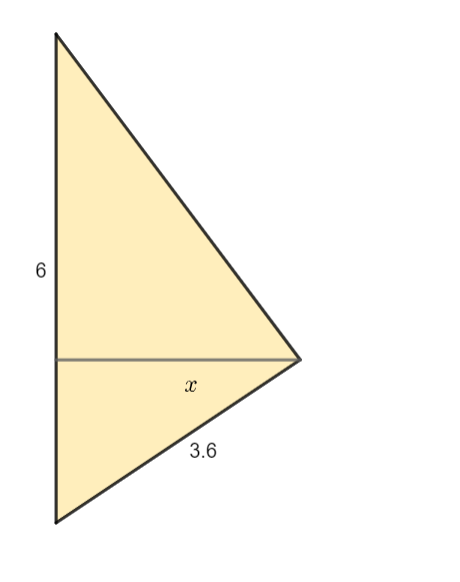Explanation: The area covered by the object would be, A=(1/2)bh.

Question 8: Calculate the area of the following right-angled triangle.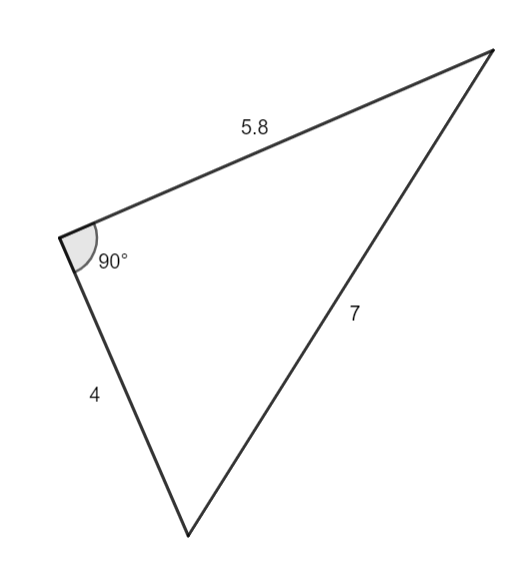Explanation: The area would be, A=(1/2)bh.

Question 9: Calculate the area of the following right-angled triangle.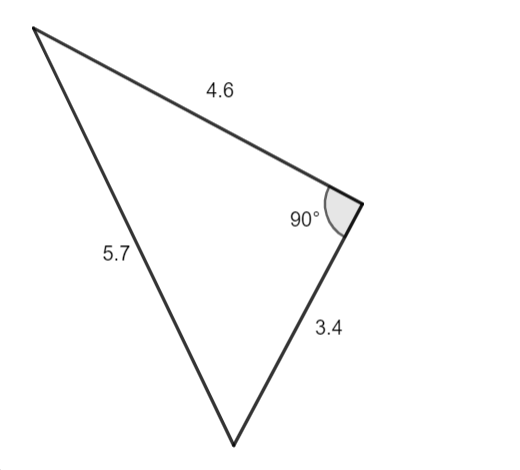Explanation: The area would be, A=(1/2)bh.

Question 10: Calculate the area of the triangle.